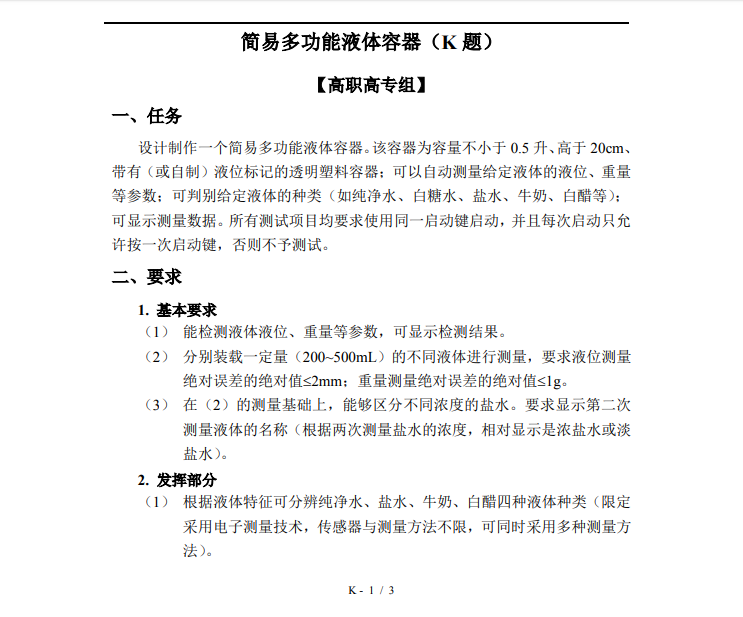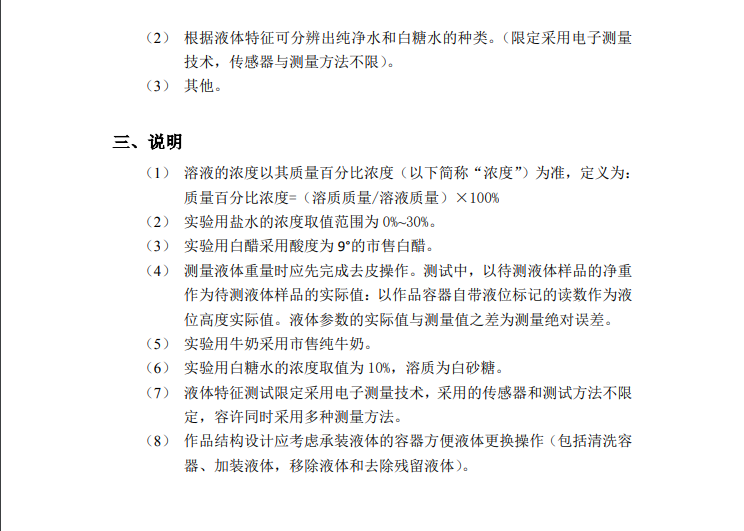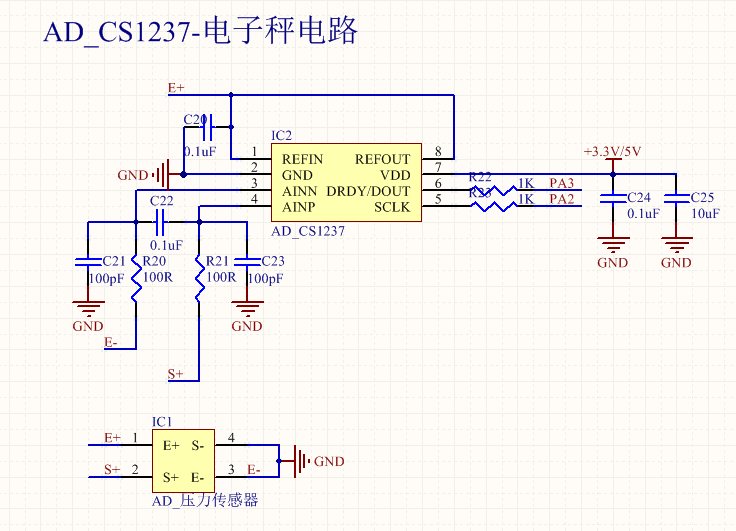• 包括cs1237技术手册、cs1237C文件H文件驱动程序，还有cs1237芯片链接原理图，谢谢大家。欢迎下载
• 电子秤是我们日常生活中常用的称重设备，广泛应用于各个行业中。电子秤在结构和原理上取代了以杠杆平衡为原理的传统机械式称量工具。相比传统的机械式称量工具，电子...电子秤主控芯片由低功耗模数转换芯片CS1237及R...
电子秤是我们日常生活中常用的称重设备，广泛应用于各个行业中。电子秤在结构和原理上取代了以杠杆平衡为原理的传统机械式称量工具。相比传统的机械式称量工具，电子秤具有称量精度高、装机体积小、应用范围广、易于操作使用等优点，在外形布局、工作原理、结构和材料上都是全新的计量衡器。
电子秤的核心结构由称重传感器、称重模块、按键和显示模块、主控芯片等组成。
电子秤主控芯片由低功耗模数转换芯片CS1237及RISC架构MCU CSU8RF3422组合实现电子秤单片机软件开发功能。
CS1237是高精度、低功耗模数转换芯片，一路差分输入通道，带Power down功能，内置温度传感器和高精度振荡器。MCU可以通过2线的SPI 接口SCLK、DRDY与CS1237进行通信，对其进行配置，例如通道选择、PGA选择、输出速率选择 等。
下面主要讲下关于CS1237对于电子秤的技术设计：
硬件设计
通讯时序
CS1237采用2线的通讯接口：DRDY/DOUT（数据线）、SCLK（时钟线）。当DRDY拉低后，表明数据已经转换完成，输入的第1个SCLK就可以将输出的最高位读出，在24个SCLK后，将所有的24位DOUT数据读出。如果需要更改配置（通道、PGA、速率）或关闭传感器激励源以节省功耗，可以通过相应的命令对CS1237的寄存器进行配置。
软件处理
由于传感器的温度特性有时候会出现偏差，需要补偿才能达到性能要求。此时可以利用CS1237内置的温度传感器读取温度值，用软件的方式来分段补偿，以节省成本。 　　当电子秤处于闲置状态，可以通过间歇开启/关闭传感器的方式来节省系统功耗，如果有重物放置，再恢复到正常的状态。
展开全文• 高精度CS1237芯片 主要功能特点 内置晶振 集成温度传感器 带Power down功能 2线SPI接口，最快速率为1.1MHz ADC功能特点 24位无失码 PGA放大倍数可选：1、2、64、128 1路24位无失码的差分输入，在PGA=128...
电子秤凭借着其性能稳定、直观便利等特点，已经在各个领域取代机械秤的地位。但随着技术的发展，人们对其性能有着更高的要求，比如：精度更高、功耗更小、成本更低。 本文介绍了24位高精度ADC芯片——CS1237应用在电子秤上的解决方案。系统通过CS1237内置放大器对称重传感器的微弱电压信号进行放大，然后通过Sigma-Delta ADC进行模数转换，最后使用2线SPI接口输出数据给MCU来进行处理。MCU处理完毕后，把重量信息送往显示模块或无线发射模块。 一、系统设计 电子秤由CS1237、称重传感器、MCU、按键模块、显示模块等组成（见下图）。
2.2、通讯时序 CS1237采用2线的通讯接口：DRDY/DOUT（数据线）、SCLK（时钟线）。当DRDY拉低后，表明数据已经转换完成，输入的第1个SCLK 就可以将输出的最高位读出，在24个SCLK后，将所有的24位DOUT数据读出。如果需要更改配置（通道、PGA、速率）或关闭传感器激励源以节省功耗，可以通过相应的命令对CS1237的寄存器进行配置。
由于传感器的温度特性往往不是很理想，需要补偿才能达到性能要求。此时可以利用CS1237内置的温度传感器读取温度值，用软件的方式来分段补偿，以节省成本。 当电子秤处于闲置状态，可以通过间歇开启/关闭传感器的方式来节省系统功耗，如果有重物放置，再恢复到正常的状态。 三、总结 CS1237具有小体积（SOP8封装）、集成度高、外围电路简单等特点，可以使得整个PCB非常小。从而在保证性能满足需求的同时，可以有效降低生产厂家的BOM成本。其有效位可达20位以上，对于典型的2mV/V称重传感器可以实现60,000d以下的分辨率，满足现有大部分电子秤的精度需求。用户还可以利用CS1237的传感器激励源开关在电子秤休眠时关闭称重传感器的电源，以节省功耗，大大延长蓄电池的使用时间。同时，CS1237的2线SPI通讯接口可以简化主控的软硬件开销，使得用户可以选择成本更低的MCU。
展开全文电子秤方案
• STM32F103 用CS1237 /HX711 芯片制作电子秤 ** 【注：学习STM32总结做的笔记，大神勿喷。有不足之处还望不吝赐教，谢谢。工程代码在最后。】 19年全国大学生电子设计大赛 简易多功能液体容器电子秤 CS1237 HX711 ...
STM32F103 用CS1237 /HX711 芯片制作电子秤
【注：学习STM32总结做的笔记，大神勿喷。有不足之处还望不吝赐教，谢谢。工程代码在最后。】
19年全国大学生电子设计大赛 简易多功能液体容器电子秤 CS1237 HX711 压力传感器 STM32F103 2019年全国大学生电子设计竞赛试题清单 2019年全国大学生电子设计竞赛仪器设备和主要元器件清单

【福利/参考/学习资料】 2019年全国大学生电子设计竞赛仪器设备和主要元器件清单 2019年全国大学生电子设计竞赛试题清单 2017年全国大学生电子设计竞赛仪器设备和主要元器件清单 全国大学生电子设计竞赛资料（收集） https://pan.baidu.com/s/1Ayy-kWOaLqlpOQgsYy7UUw https://pan.baidu.com/s/1fhTiDL7GUUBYx-WcJoWHNQ https://pan.baidu.com/s/1–VqCzF2nMporwkkKYIrXw https://pan.baidu.com/s/1eIk9hmbHhbBSZ4tW1o1T7Q （文件太多，只能分开）
本人有幸参与了19年的全国大学生电子设计大赛（广东赛区选拔赛），选择了K题，制作简易多功能液体容器，（即，电子秤与及检测重量、分辨不同液体和高度、密度等物理量）。电子秤制作原理什么的就不多介绍了，下面链接是电子秤制作原理，想具体了解的，可以看看。 http://www.doc88.com/p-695275844769.html 这比赛呢，我们团队使用超声波测高、电子秤测重、PH计测酸、电导计测电导，从物理、化学的角度考虑，通过不同传感器检测不同液体AD值，从而区分不同液体，具体怎么做就不细说了。。

本篇就只简单介绍STM32F103 用CS1237 /HX711 芯片制作电子秤，比赛其他的就不涉及了。
直接上原理图：mian.c
#include "stm32f10x.h"
#include "uart.h"
#include "cs1237.h"
#include "key.h"

/*
2019.8.12 子非鱼
测重，用 CS1237 和 HX711 芯片，其实做法一样，只要数据稳定，都能精准检测。程序写法都一样的。
*/

float one_weight=0,two_weight,weight,zhun_weight,zhongliang;		//重量

int main(void)
{
int i;

USART1_Init(); // 初始化串口1 波特率9600
CS1237_Init_JX();
delay_ms(300);
CS1237_Config();
delay_ms(100);

/*第一次取值 用作基准值 用于复位后去皮用 我比赛时没用到这段*/

//	for(i=0;i<20;i++)  /* 用于复位后 循环取第19次值 前面的值用于稳定数据 */
//	{
//		weight = weight + 28000; /* +28000 是因为传感器/芯片的原因导致读回来的值，是负值（-20500~正数++）。需要制零（尽可能接近零），方便从零开始计数。也有些是往负数++ ，这需要看情况。 */
//		if(i==19)
//		{
//		one_weight = weight;
//		}
//	//  printf("*******%f/r/n  ",one_weight);
//	}
//	delay_ms(100);

while (1)
{
///
/*
实时按键去皮（清零）
跟上面注释的说明一样的，现在只是实时按键清零而已
*/
if(KEY1==0)
{
delay_ms(10);
one_weight = one_weight + 28000;
}
/

two_weight = two_weight + 28000;//取一次值，处理一次
two_weight = two_weight - one_weight;	/* one_weight定义时值要为零，否则会出错 */
weight     = two_weight/100000; //这里对读取CS1237的数据 缩小了100000倍（可以缩小，也可以不缩小。我的缩小了）

//		printf("*******%f/r/n  ",weight);

/

/*
下面采用数据分段计算的方法，以求更高的精度

50                             0.210                            0.0042
100                            0.420                            0.0042
150                            0.629                            0.00419
200                            0.833                            0.004165
250                            1.044                            0.004176
300                            1.253                            0.004177
...                            ...                              ...
900                            3.766                            0.004184
*/

/*
转化为克
zhongliang = weight / c

判断读取CS1237的数据weight的值，落在哪个区间，然后选取相应的 系数c ( ad/g ) 值
*/

if(weight>0.001&&weight<0.2101){zhongliang = weight / 0.0042  ;} //50g
if(weight>0.210&&weight<0.4201){zhongliang = weight / 0.0042  ;} //100g
if(weight>0.420&&weight<0.6291){zhongliang = weight / 0.00419 ;} //150g
if(weight>0.629&&weight<1.8331){zhongliang = weight / 0.004165 ;} //200g
if(weight>0.833&&weight<1.0441){zhongliang = weight / 0.004176 ;} //250g
if(weight>1.044&&weight<1.2531){zhongliang = weight / 0.004177 ;} //300g
if(weight>1.253&&weight<1.4641){zhongliang = weight / 0.004183 ;} //350g
if(weight>1.464&&weight<1.6661){zhongliang = weight / 0.004165 ;} //400g
if(weight>1.666&&weight<1.8741){zhongliang = weight / 0.004164 ;} //450g
if(weight>1.874&&weight<2.0851){zhongliang = weight / 0.00417 ;} //500g
if(weight>2.085&&weight<2.2961){zhongliang = weight / 0.0041745 ;} //550g
if(weight>2.296&&weight<2.5071){zhongliang = weight / 0.00418 ;} //600g
if(weight>2.507&&weight<2.7171){zhongliang = weight / 0.00418 ;} //650g
if(weight>2.717&&weight<2.9241){zhongliang = weight / 0.004178 ;} //700g
if(weight>2.924&&weight<3.1381){zhongliang = weight / 0.004175 ;} //750g
if(weight>3.138&&weight<3.3481){zhongliang = weight / 0.004185 ;} //800g
if(weight>3.348&&weight<3.5561){zhongliang = weight / 0.0041835 ;} //850g
if(weight>3.556&&weight<3.7661){zhongliang = weight / 0.0041844 ;} //900g
if(weight>3.766)               {zhongliang = weight / 0.004191 ;} //900g以上

printf("*******%f*********%f/r/n  ",weight,zhongliang);
}

}

**
cs1237.c
**
#include "cs1237.h"
#include "uart.h"

//OUT引脚输入输出 方向设置  PA3
#define OUT_IN()  {GPIOA->CRL&=0XFFFF0FFF;GPIOA->CRL|=8<<12;}
#define OUT_OUT() {GPIOA->CRL&=0XFFFF0FFF;GPIOA->CRL|=3<<12;}

// PA2-----CLK
// PA3-----OUT

void Delay1us(void)
{
__IO uint32_t t=5;

while(t--);
}

void Delay1ms(void)
{
__IO uint32_t t=8000;

while(t--);
}

void delay_ms(__IO uint16_t ms)
{
do{
Delay1ms();
}while(ms--);
}

// 初始化PA2 PA3
// PA2-----CLK
// PA3-----OUT
void CS1237_Init_JX(void)
{
GPIO_InitTypeDef  GPIO_InitStructure;

RCC_APB2PeriphClockCmd(RCC_APB2Periph_GPIOA, ENABLE);

// PA2 ----- CLK  设置为输出
// PA3 ----- OUT  设置为输出
GPIO_InitStructure.GPIO_Pin = GPIO_Pin_2 | GPIO_Pin_3;
GPIO_InitStructure.GPIO_Mode = GPIO_Mode_Out_PP;
GPIO_InitStructure.GPIO_Speed = GPIO_Speed_50MHz;
GPIO_Init(GPIOA, &GPIO_InitStructure);

GPIO_SetBits(GPIOA, GPIO_Pin_2);	// CLK拉高
GPIO_SetBits(GPIOA, GPIO_Pin_3);	// OUT拉高
}

//配置CS1237芯片
void CS1237_Config(void)
{
unsigned char i;
unsigned char dat;
unsigned int count_i=0;//溢出计时器

dat = 0X0C;   //芯片地配置 内部REF 输出40HZ PGA=128 通道A 0X1C
OUT_OUT();
GPIO_SetBits(GPIOA, GPIO_Pin_3); //OUT引脚拉高
OUT_IN();
GPIO_ResetBits(GPIOA, GPIO_Pin_2);// 时钟拉低
{
delay_ms(1);
count_i++;
if(count_i > 300)
{
OUT_OUT();
GPIO_SetBits(GPIOA, GPIO_Pin_3); // OUT引脚拉高
GPIO_SetBits(GPIOA, GPIO_Pin_2); // CLK引脚拉高
return;//超时，则直接退出程序
}
}
for(i=0;i<29;i++)// 1 - 29
{
GPIO_SetBits(GPIOA, GPIO_Pin_2);	// CLK=1;
Delay1us();
GPIO_ResetBits(GPIOA, GPIO_Pin_2);	// CLK=0;
Delay1us();
}
OUT_OUT();
GPIO_SetBits(GPIOA, GPIO_Pin_2);Delay1us();GPIO_SetBits(GPIOA, GPIO_Pin_3);GPIO_ResetBits(GPIOA, GPIO_Pin_2);Delay1us();//30
GPIO_SetBits(GPIOA, GPIO_Pin_2);Delay1us();GPIO_SetBits(GPIOA, GPIO_Pin_3);GPIO_ResetBits(GPIOA, GPIO_Pin_2);Delay1us();//31
GPIO_SetBits(GPIOA, GPIO_Pin_2);Delay1us();GPIO_ResetBits(GPIOA, GPIO_Pin_3);GPIO_ResetBits(GPIOA, GPIO_Pin_2);Delay1us();//32
GPIO_SetBits(GPIOA, GPIO_Pin_2);Delay1us();GPIO_ResetBits(GPIOA, GPIO_Pin_3);GPIO_ResetBits(GPIOA, GPIO_Pin_2);Delay1us();//33
GPIO_SetBits(GPIOA, GPIO_Pin_2);Delay1us();GPIO_SetBits(GPIOA, GPIO_Pin_3);GPIO_ResetBits(GPIOA, GPIO_Pin_2);Delay1us();//34
GPIO_SetBits(GPIOA, GPIO_Pin_2);Delay1us();GPIO_ResetBits(GPIOA, GPIO_Pin_3);GPIO_ResetBits(GPIOA, GPIO_Pin_2);Delay1us();//35
GPIO_SetBits(GPIOA, GPIO_Pin_2);Delay1us();GPIO_SetBits(GPIOA, GPIO_Pin_3);GPIO_ResetBits(GPIOA, GPIO_Pin_2);Delay1us();//36
//37     写入了0x65
GPIO_SetBits(GPIOA, GPIO_Pin_2);	// CLK=1;
Delay1us();
GPIO_ResetBits(GPIOA, GPIO_Pin_2);	// CLK=0;
Delay1us();

for(i=0;i<8;i++)// 38 - 45个脉冲了，写8位数据
{
GPIO_SetBits(GPIOA, GPIO_Pin_2);	// CLK=1;
Delay1us();
if(dat&0x80)
GPIO_SetBits(GPIOA, GPIO_Pin_3);// OUT = 1
else
GPIO_ResetBits(GPIOA, GPIO_Pin_3);
dat <<= 1;
GPIO_ResetBits(GPIOA, GPIO_Pin_2);	// CLK=0;
Delay1us();
}
GPIO_SetBits(GPIOA, GPIO_Pin_3);// OUT = 1
GPIO_SetBits(GPIOA, GPIO_Pin_2);	// CLK=1;
Delay1us();
GPIO_ResetBits(GPIOA, GPIO_Pin_2);	// CLK=0;
Delay1us();
}

// 读取芯片的配置数据
{
unsigned char i;
unsigned char dat=0;//读取到的数据
unsigned int count_i=0;//溢出计时器
//	unsigned char k=0,j=0;//中间变量

OUT_OUT();
GPIO_SetBits(GPIOA, GPIO_Pin_3); //OUT引脚拉高
OUT_IN();
GPIO_ResetBits(GPIOA, GPIO_Pin_2);//时钟拉低
{
delay_ms(1);
count_i++;
if(count_i > 300)
{
OUT_OUT();
GPIO_SetBits(GPIOA, GPIO_Pin_2);	// CLK=1;
GPIO_SetBits(GPIOA, GPIO_Pin_3);	// OUT=1;
return 1;//超时，则直接退出程序
}
}

for(i=0;i<29;i++)// 产生第1到29个时钟
{
GPIO_SetBits(GPIOA, GPIO_Pin_2);	// CLK=1;
Delay1us();
GPIO_ResetBits(GPIOA, GPIO_Pin_2);	// CLK=0;
Delay1us();
}

OUT_OUT();

GPIO_SetBits(GPIOA, GPIO_Pin_2); // CLK=1;
Delay1us();
GPIO_SetBits(GPIOA, GPIO_Pin_3);
GPIO_ResetBits(GPIOA, GPIO_Pin_2); // CLK=0;
Delay1us();// 这是第30个时钟

GPIO_SetBits(GPIOA, GPIO_Pin_2); // CLK=1;
Delay1us();
GPIO_ResetBits(GPIOA, GPIO_Pin_3);
GPIO_ResetBits(GPIOA, GPIO_Pin_2); // CLK=0;
Delay1us();// 这是第31个时钟

GPIO_SetBits(GPIOA, GPIO_Pin_2); // CLK=1;
Delay1us();
GPIO_SetBits(GPIOA, GPIO_Pin_3);
GPIO_ResetBits(GPIOA, GPIO_Pin_2); // CLK=0;
Delay1us();//32

GPIO_SetBits(GPIOA, GPIO_Pin_2); // CLK=1;
Delay1us();
GPIO_ResetBits(GPIOA, GPIO_Pin_3);
GPIO_ResetBits(GPIOA, GPIO_Pin_2); // CLK=0;
Delay1us();//33

GPIO_SetBits(GPIOA, GPIO_Pin_2); // CLK=1;
Delay1us();
GPIO_SetBits(GPIOA, GPIO_Pin_3);
GPIO_ResetBits(GPIOA, GPIO_Pin_2); // CLK=0;
Delay1us();//34

GPIO_SetBits(GPIOA, GPIO_Pin_2); // CLK=1;
Delay1us();
GPIO_SetBits(GPIOA, GPIO_Pin_3);
GPIO_ResetBits(GPIOA, GPIO_Pin_2); // CLK=0;
Delay1us();//35

GPIO_SetBits(GPIOA, GPIO_Pin_2); // CLK=1;
Delay1us();
GPIO_ResetBits(GPIOA, GPIO_Pin_3);
GPIO_ResetBits(GPIOA, GPIO_Pin_2); // CLK=0;
Delay1us();//36

GPIO_SetBits(GPIOA, GPIO_Pin_3);
GPIO_SetBits(GPIOA, GPIO_Pin_2);	// CLK=1;
Delay1us();
GPIO_ResetBits(GPIOA, GPIO_Pin_2);	// CLK=0;
Delay1us();//37     写入0x56 即读命令

dat=0;
OUT_IN();
for(i=0;i<8;i++)// 第38 - 45个脉冲了，读取数据
{
GPIO_SetBits(GPIOA, GPIO_Pin_2);	// CLK=1;
Delay1us();
GPIO_ResetBits(GPIOA, GPIO_Pin_2);	// CLK=0;
Delay1us();
dat <<= 1;
dat++;
}

//第46个脉冲
GPIO_SetBits(GPIOA, GPIO_Pin_2);	// CLK=1;
Delay1us();
GPIO_ResetBits(GPIOA, GPIO_Pin_2);	// CLK=0;
Delay1us();

OUT_OUT();
GPIO_SetBits(GPIOA, GPIO_Pin_3); //OUT引脚拉高

return dat;
}

{
unsigned char i;
uint32_t dat=0;//读取到的数据
unsigned int count_i=0;//溢出计时器
int32_t temp;

OUT_OUT();
GPIO_SetBits(GPIOA, GPIO_Pin_3); //OUT引脚拉高
OUT_IN();
GPIO_ResetBits(GPIOA, GPIO_Pin_2);//时钟拉低
{
delay_ms(1);
count_i++;
if(count_i > 300)
{
OUT_OUT();
GPIO_SetBits(GPIOA, GPIO_Pin_2);	// CLK=1;
GPIO_SetBits(GPIOA, GPIO_Pin_3);	// OUT=1;
return 1;//超时，则直接退出程序
}
}

dat=0;
for(i=0;i<24;i++)//获取24位有效转换
{
GPIO_SetBits(GPIOA, GPIO_Pin_2);	// CLK=1;
Delay1us();
dat <<= 1;
dat ++;
GPIO_ResetBits(GPIOA, GPIO_Pin_2);	// CLK=0;
Delay1us();
}

for(i=0;i<3;i++)//接着前面的时钟 再来3个时钟
{
GPIO_SetBits(GPIOA, GPIO_Pin_2);	// CLK=1;
Delay1us();
GPIO_ResetBits(GPIOA, GPIO_Pin_2);	// CLK=0;
Delay1us();
}

OUT_OUT();
GPIO_SetBits(GPIOA, GPIO_Pin_3); // OUT = 1;

if(dat&0x00800000)// 判断是负数 最高位24位是符号位
{
temp=-(((~dat)&0x007FFFFF) + 1);// 补码变源码
}
else
temp=dat; // 正数的补码就是源码

return temp;
}



cs1237.h

#ifndef __CS1237_H
#define __CS1237_H

#include "stm32f10x.h"

void Delay1us(void);
void Delay1ms(void);
void delay_ms(__IO uint16_t ms);
void CS1237_Init_JX(void);
void CS1237_Config(void);

#endif


【注：文件源码是Keil uVision5–STM32工程文件】 参考文件源码下载链接： https://download.csdn.net/download/qq_28056277/11541590 原创文章，转载请注明出处，谢谢。
展开全文STM32F103 HX711
• 电子秤是我们日常生活中常用的称重设备，广泛应用于各个行业中。电子秤在结构和原理上取代了以杠杆平衡为原理的传统机械式称量工具。相比传统的机械式称量工具，电子...电子秤主控芯片由低功耗模数转换芯片CS1237及R...
电子秤是我们日常生活中常用的称重设备，广泛应用于各个行业中。电子秤在结构和原理上取代了以杠杆平衡为原理的传统机械式称量工具。相比传统的机械式称量工具，电子秤具有称量精度高、装机体积小、应用范围广、易于操作使用等优点，在外形布局、工作原理、结构和材料上都是全新的计量衡器。
电子秤的核心结构由称重传感器、称重模块、按键和显示模块、主控芯片等组成。
电子秤主控芯片由低功耗模数转换芯片CS1237及RISC架构MCU CSU8RF3422组合实现电子秤单片机软件开发功能。
CS1237是高精度、低功耗模数转换芯片，一路差分输入通道，带Power down功能，内置温度传感器和高精度振荡器。MCU可以通过2线的SPI 接口SCLK、DRDY与CS1237进行通信，对其进行配置，例如通道选择、PGA选择、输出速率选择 等。
下面主要讲下关于CS1237对于电子秤的技术设计：
硬件设计
通讯时序
CS1237采用2线的通讯接口：DRDY/DOUT（数据线）、SCLK（时钟线）。当DRDY拉低后，表明数据已经转换完成，输入的第1个SCLK就可以将输出的最高位读出，在24个SCLK后，将所有的24位DOUT数据读出。如果需要更改配置（通道、PGA、速率）或关闭传感器激励源以节省功耗，可以通过相应的命令对CS1237的寄存器进行配置。
软件处理
由于传感器的温度特性有时候会出现偏差，需要补偿才能达到性能要求。此时可以利用CS1237内置的温度传感器读取温度值，用软件的方式来分段补偿，以节省成本。 　　当电子秤处于闲置状态，可以通过间歇开启/关闭传感器的方式来节省系统功耗，如果有重物放置，再恢复到正常的状态。
展开全文• CS1237 是一款高精度、低功耗模数转换芯片， 一路差分输入通道，内置温度传感器和 高精度振荡器。 CS1237 的 PGA 可选： 1、 2、 64、 128，默认为 128。 CS1237 正常模式下的 ADC 数据输出速率可选： 10Hz、 40Hz、...

万次阅读 热门讨论 2018-06-01 22:43:12STM32 电子设计 嵌入式
• 这是一个采集称重传感器的程序，采集芯片CS1237，提供大家参考
• STM32的CS1237的驱动程序，已在STM32L071单片机上进行过实际测试。使用通用的hal库进行的初始化以及采集过程，程序内容比较简练，包括有初始化函数，设置函数，读取值函数，读取设置函数，以及平均采集5次用的函数，...电子设计电子设计
• gcc -fPIC CS1237.c -o CS1237.so -shared -I/usr/include/python2.7 -I/usr/lib/python2.7/config 然后在此目录下新建一个test.py验证一下： # test.py # coding=utf-8 import CS1237 tmp = CS1302....python git c语言
• cs1237-AD芯片做采集选用128倍增益 用 modbus进行通讯地址0x0000 0x0001做数据高低位 用的 新唐003单片机
• STM32F103 用CS1237 /HX711 芯片制作电子秤 具体可参考： https://blog.csdn.net/qq_28056277/article/details/99445088
• CS5268芯片数据手册，CS5268规格书，CS5268方案应用，CS5268说明书
• cs5463是一款电量测量芯片，测量精度很高，电路简单。提供了电量测量芯片的驱动
• STM32F103 用CS1237 /HX711 芯片制作电子秤 具体可参考： https://blog.csdn.net/qq_28056277/article/details/99445088
• CS7123芯片是深圳市芯海科技有限公司自主设计的高速/高精度视频DAC芯片，其内部包括三路10位电流导引(Current Steering)结构的DAC，最大采样速度达到240MHz。CS7123结构框图见图1，它包括三路高速、10位输入的视频DA...
• Arduino库/ ESP，用于与Cirrus Logic CS5490芯片进行通信 请考虑阅读 其他贡献者 安东尼奥·塞萨尔·德·卡斯特罗·利马 安德烈·库恩（AndréKuhn） 维陶塔斯·加布留纳斯（Vytautas Gabriunas）
• 基于CS5463芯片的轨道车辆能耗计量装置.pdf...